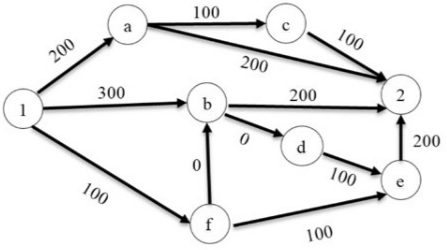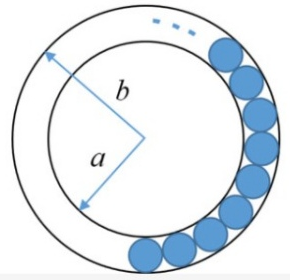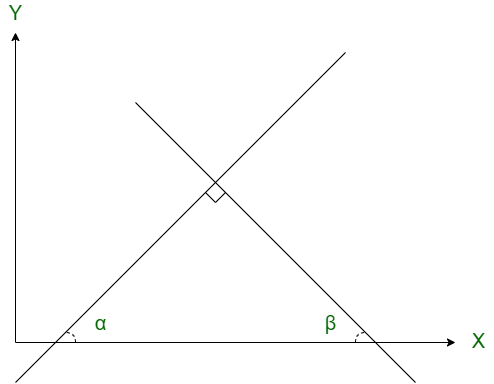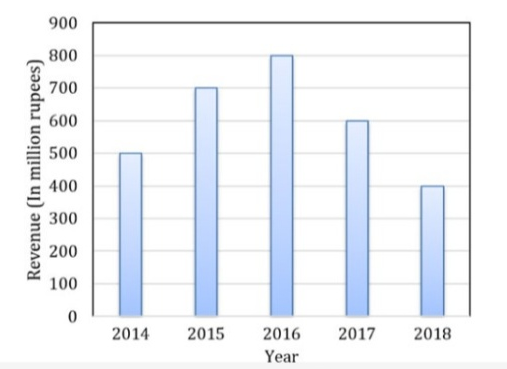# GATE CS 2020

• Last Updated : 29 Oct, 2021

 Question 1
Raman is confident of speaking English _______ six months as he has been practising regularly _______ the last three weeks.
 A during, for B for, since C for, in D within, for
GATE CS 2020    English
Discuss it

Question 1 Explanation:
'Within' means ‘inside or not further than a particular area or space’. We can use within to refer to time. In ‘present perfect continuous tense’ to talk about a period of time:
• We use 'since' to refer back to a previous point in time. We use 'since' as a preposition with a date, a time or a noun phrase.
• We use 'for' with a period of time to refer to duration (how long something lasts).
After a negative we can use 'for' and 'in' with the same meaning. In is particularly common in American. We use 'in' to say how long it takes someone to do something. We use 'during' before nouns and noun phrases to refer to when something happens over a period of time. During can refer to the whole time of the event. We don’t use 'during' when we refer to numbers and lengths of time. In this case, we use 'for'. Option (D) is correct.
 Question 2
His knowledge of the subject was excellent but his classroom performance was _______ .
 A extremely poor B good C desirable D praiseworthy
GATE CS 2020    English
Discuss it

Question 2 Explanation:
'but' is used to indicate that something in the second part of the sentence will contradict something that was said in the first part of the sentence. Option (A) is correct.
 Question 3
Select the word that fits the analogy:
`Cook : Cook :: Fly : _______ `
 A Flyer B Flying C Flew D Flighter
GATE CS 2020    English
Discuss it

Question 3 Explanation:
`Verb : Noun `
One who cooks is a cook and one who flies any aircraft is a flyer. Option (A) is correct.
 Question 4

The dawn of the 21st century witnessed the melting glaciers oscillating between giving too much and too little to billions of people who depend on them for fresh water. The UN climate report estimates that without deep cuts to man-made emissions, at least 30% of the northern hemisphere’s surface permafrost could melt by the end of the century. Given this situation of imminent global exodus of billions of people displaced by rising seas, nation-states need to rethink their carbon footprint for political concerns, if not for environmental ones.

Which one of the following statements can be inferred from the given passage ?

 A Nation-states do not have environmental concerns. B Nation-states are responsible for providing fresh water to billions of people. C Billions of people are responsible for man-made emissions. D Billions of people are affected by melting glaciers.
GATE CS 2020    English    Reading Comprehension
Discuss it

Question 4 Explanation:

Option (D) clearly explains the second last line of the passage.

 Question 5
There are multiple routes to reach from node 1 to node 2, as shown in the network.The cost of travel on an edge between two nodes is given in rupees. Nodes ‘a’, ‘b’, ‘c’, ‘d’, ‘e’, and ‘f’ are toll booths. The toll price at toll booths marked ‘a’ and ‘e’ is Rs. 200, and is Rs. 100 for the other toll booths. Which is the cheapest route from node 1 to node 2 ?
 A 1−a−c−2 B 1−f−b−2 C 1−b−2 D 1−f−e−2
GATE CS 2020    General Aptitude
Discuss it

Question 5 Explanation:
(A) Cost of 1-a-c-2 :

`200 + 200 + 100 + 100 + 100 = 700`

(B) Cost of 1-f-b-2 :

`100 + 100 + 0 + 100 +200 = 500`

(C) Cost of 1-b-2 :

`300 + 100 + 200 = 600 `

(D) Cost of 1-f-e-2 :

`100 + 100 + 100 + 200 + 200 = 700`

Hence, 1-f-b-2 is having minimum cost.

Option (B) is correct.

 Question 6
Goods and Services Tax (GST) is an indirect tax introduced in India in 2017 that is imposed on the supply of goods and services, and it subsumes all indirect taxes except few. It is a destination-based tax imposed on goods and services used, and it is not imposed at the point of origin from where goods come. GST also has a few components specific to state governments, central government and Union Territories (UTs).
Which one of the following statements can be inferred from the given passage ?
 A GST is imposed on the production of goods and services. B GST includes all indirect taxes. C GST does not have a component specific to UT. D GST is imposed at the point of usage of goods and services.
GATE CS 2020    English    Reading Comprehension
Discuss it

Question 6 Explanation:
Second statement "It is a destination-based tax imposed on goods and services used, and it is not imposed at the point of origin from where goods come.". Option (D) is correct.
 Question 7

If values of P=3, R=27, T=243, then find the value of Q+S = ________ .

 A 40 B 80 C 90 D 180
GATE CS 2020    General Aptitude    Algebra
Discuss it

Question 7 Explanation:

Given, sequence is in n-th power of 3. Starting from n=1 and position 'P' alphabetically order.

```P = 31 = 3
Q = 32 = 9
R = 33 = 27
S = 34 = 81
T = 35 = 243 ```

Therefore,

`Q+S = 9+81 = 90 `

Option (C) is correct.

 Question 8
The figure below shows an annular ring with outer and inner as b and a, respectively. The annular space has been painted in the form of blue colour circles touching the outer and inner periphery of annular space. If maximum n number of circles can be painted, then the unpainted area available in annular space is _________ .A π[(b2−a2)−(n/4)(b−a)2] B π[(b2−a2)−n(b−a)2] C π[(b2−a2)+(n/4)(b−a)2] D π[(b2−a2)+n(b−a)2]
GATE CS 2020    General Aptitude    50 Aptitude & Reasoning MCQs with Answers
Discuss it

Question 8 Explanation:
Area of 1 blue circle,
`= π((b-a)/2)2`
Hence, area of n blue circles,
`= nπ((b-a)/2)2`
Now, Area of annular ring
`= πb2 – πa2 `
```= (Area of annular ring) – (Area of n blue color circles)
= ( πb2 – πa2 ) - ( nπ((b-a)/2)2 )
= π( b2 – a2 ) - π( (n/4)(b-a)2 )
= π[(b2−a2)−(n/4)(b−a)2] ```
Option (A) is correct.
 Question 9
Two straight lines are drawn perpendicular to each other in X−Y plane. If α and β are the acute angles the straight lines make with the X- axis, then α+β is ________ .
 A 60° B 90° C 120° D 180°
GATE CS 2020    General Aptitude
Discuss it

Question 9 Explanation:
According to given question,Now, as we know that, in a triangle sum of internal angles is equal to 180°. That is,
```→ ∠a + ∠b + ∠c = 180°
→ α + β + 90° = 180°
→ α + β = 180° - 90° = 90°
→ α + β = 90°```
Option (B) is correct.
 Question 10
The total revenue of a company during 2014−2018 is shown in the bar graph. If the total expenditure of the company in each year is 500 million rupees, then the aggregate profit or loss (in percentage) on the total expenditure of the company during 2014−2018 is _________ .A 16.67% profit B 16.67% loss C 20% profit D 20% loss
GATE CS 2020    General Aptitude    Probability
Discuss it

Question 10 Explanation:
Bar-graph: 2014-2018, Total expenditure,
```= 500 million/year
= 500 × 5
= 2500 million ```
Now, Revenue total (from the graph),
```= 400 + 500 + 600 + 700 + 800
= 3000 million ```
Therefore, Profit,
`= 3000 - 2500 = 500 `
Profit (in percentage),
```= (500/2500)*100 %
= 20 % profit ```
Option (C) is correct.
There are 65 questions to complete.
My Personal Notes arrow_drop_up